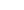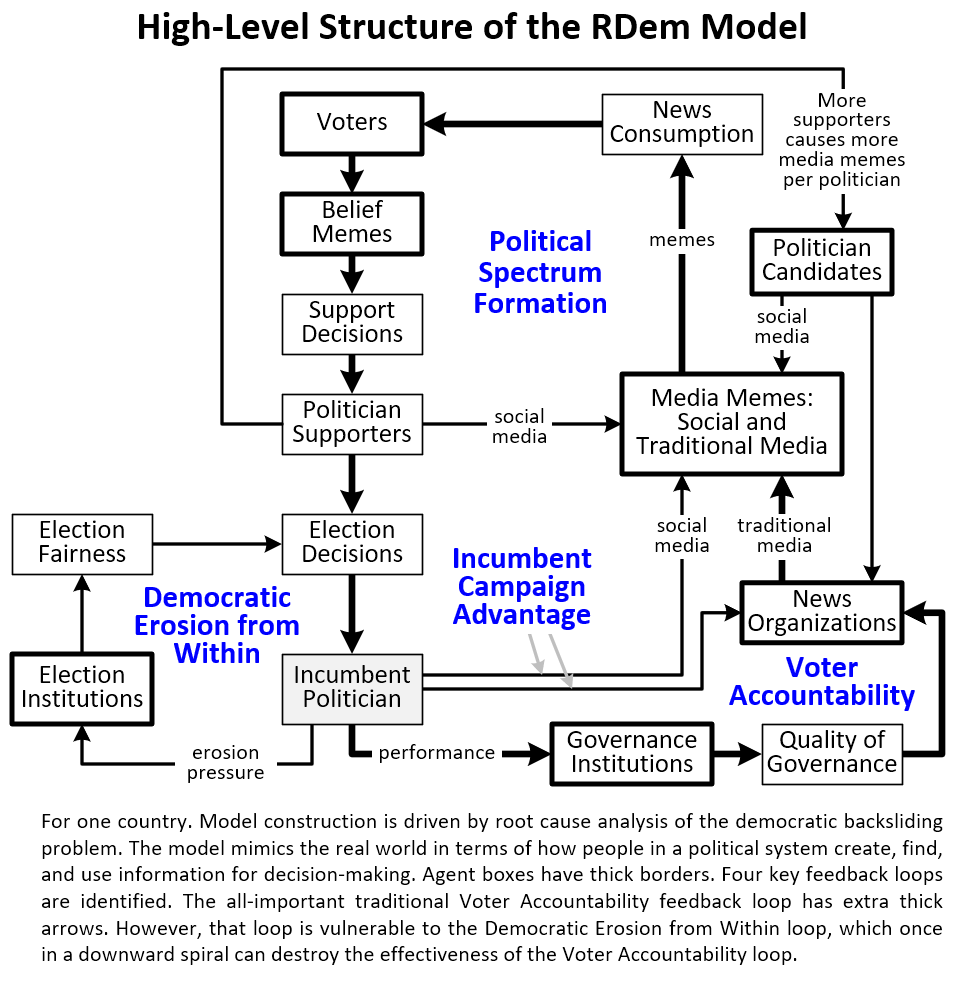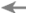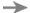# Agent-Based Modeling

Agent-Based Modeling (ABM) is simulation modeling that centers on modeling the behavior of agents. An agent is an autonomous entity of some kind that interacts with its environment and other agents, and makes rule-based decisions. It is these decisions that cause the dynamic behavior of the model over time.

Examples of agents are voters, countries, and birds. An ABM of bird flight would use simple decision rules to show how bird flocks behaved. If the model was built with reuse in mind, the model could be reused to explore the behavior of schools of fish by replacing the bird agents with fish agents.

## The essential characteristics of an ABM

There are millions of ways to design an ABM. What they all have in common is:

1. Agents who interact with other agents and the environment and make rule-based decisions.

2. Visual output in the form of graphs or other things like agents moving on a surface.

3. Scenarios that show how the agents and related data behave when the model is run.

4. Settings for the model. Changing a setting makes the model run differently, unless the setting has no effect. Each scenario consists of a collection of settings that define that scenario, such as the goal of an agent and what decision rule parameters the agent uses.

## When to use ABM

The three main families of modeling are based on agents (ABM), feedback loops (system dynamics), and mathematical equations (econometric modeling, physics models, weather models, industrial processes, etc.) The third is specialialized. The first two are generalized, which explains their power.

If you have a novel problem type that needs modeling to gain deeper understanding of what affects problem behavior, and you can use ABM, system dynamics, or a combination of the two, then right away you have an advantage over the third family. The advantage is there is already a large amount of reusable concepts and tools you can use to build your model. This can increase productivity by an order of magnitude or more.

If it's not a novel problem type, then specialized tools in the third family have already been developed.

The leading tools for building an ABM are AnyLogic (expensive at \$4000) and NetLogo (free, commonly used in college courses on ABM). A new tool in its early stages that combines ABM and system dynamics is Ventity. Once how ABMs work is understood, you can also program your own ABM model.

An ABM is preferred over the other two modeling families when:

1. The central behavior to understand is the behavior of agents.

2. The agents have 3 or more properties that differentiate the agents. For example, if you are modeling banks, a bank has customers, deposits, interest rates, loan rates, and so on. That is best modeling with ABM. But if you are modeling natural resource depletion and each resource has only starting amount and use rate, then a feedback loop modeling approach would be best.

3. The agents follow distinct rules of behavior that need to be deeply understood.

4. Important emergent behavior appears due to agent interaction.

However, solving difficult dynamic system problems that center on agent behavior also requires understanding the feedback loops involved. This is because of the fundamental axiom of system dynamics: The behavior of a system arises from the structure of its feedback loops. Here we can recommend the strategy of:

1. First model the overall feedback loop structure of the problem, using causal loop diagrams and/or system dynamics.

2. Let the feedback loop model drive design and construction of the ABM.

We have used this strategy ourself on the democratic backsliding problem, using this causal flow diagram:As we constructed the diagram we identified the agents. These were news organizations, media memes, belief memes, voters, and politicians.

Browse the GlossaryPreviousNextRELATED INFORMATION
Wikipedia

For an in depth description of ABM, please see the Wikipedia entry on Agent-based Model. This begins with:

"An agent-based model (ABM) is a computational model for simulating the actions and interactions of autonomous agents (both individual or collective entities such as organizations or groups) in order to understand the behavior of a system and what governs its outcomes."